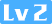1 手写ArrayList核心源码九路393 1 1

## 手写ArrayList核心源码

ArrayList是Java中常用的数据结构，不光有ArrayList，还有LinkedList,HashMap,LinkedHashMap,HashSet,Queue，PriorityQueue等等，我们将手写这些常用的数据结构的核心源码，用尽量少的代码来揭示核心原理。

public class QList<T> {
//默认的数组的大小
private final int DEFAULT_LIST_SIZE = 8;

//存放数据的地方
private Object[] mData;

//下一个可以存放数据的当前数组的索引
private int mSize;

......
}    

public class QArrayList<T> {
//默认的数组的大小
private final int DEFAULT_LIST_SIZE = 8;

//存放数据的地方
private Object[] mData;

//下一个可以存放数据的当前数组的索引
private int mSize;

public QArrayList() {
//new 一个数组，用来存放
mData = new Object[DEFAULT_LIST_SIZE];

//下一个可以存放数据的当前数组的索引为0
mSize = 0;
}

public QArrayList(int capacity){
if(capacity <= 0 || capacity > Integer.MAX_VALUE){
throw new RuntimeException("invalid capacity");
}

mData = new Object[capacity];
mSize = 0;
}

//返回当时数组的已经存放了多少个元素
public int size() {
return mSize;
}

//返回数组的总大小，其实这个接口没有必要对外提供，这里我们只是为了演示用
public int capacity() {
return mData.length;
}

//添加一个元素
public void add(T e) {
//规定不允许添加一个空元素
if(e == null){
return;
}

//如果当前数组已经满了，扩容为原来数组的2倍
if (mSize >= mData.length) {

//扩容
resize();
}

//将添加的元素添加到数组中
mData[mSize] = e;

//同时 mSize++ 指向下一个可以存放数据的位置
mSize++;
}

//获取指定位置的元素，如果position不合法，直接抛出异常
//这样做是有必要的，我们提供的是一个库
// 直接抛出异常让使用知道用错了，没有必要 return null
// 因为这是个库，不是业务，就算return null，也是业务层的事
public T get(int position) {
if (position < 0 || position >= mData.length) {
throw new RuntimeException("position is invalid");
}

// position 大于 mSize 也没有关系，因为也是返回null，证明没有获取到
return (T) mData[position];
}

//删除指定位置的元素
public T remove(int position) {
//和上面一样，位置不合法直接抛出异常
if (position < 0 || position >= mData.length) {
throw new RuntimeException("position is invalid");
}

//把当前要删除的元素保存下来，最后返回要删除的元素
T e = (T) mData[position];

//删除后，把后面的所有元素都往前移位
for (int i = position + 1; i < mData.length; i++) {
mData[i - 1] = mData[i];
}

//别忘了 mSize 要 --
mSize--;

//返回删除的元素
return e;
}

//删除指定的元素
public boolean remove(T e) {
//因为数组可能没有满，如果删除的是null，没有必要，我们不允许
if (e == null) {
return false;
}

//找到删除元素的位置
int position = -1;
for (int i = 0; i < mData.length; i++) {
if (e == mData[i] || e.equals(mData[i])) {
position = i;
break;
}
}

//没有找到就返回
if (position == -1) {
return false;
}

//删除
return remove(position) != null;
}

//扩容,我们都以2倍的容量扩容
private void resize() {
Object[] old = mData;
mData = new Object[mData.length * 2];
for (int i = 0; i < old.length; i++) {
mData[i] = old[i];
}

old = null;
}
}


    public static void main(String[] args) {
QArrayList<String> list = new QArrayList<>();
list.add("tom");
list.add("jim");
list.add("lilei");
list.add("hanmeimei");

System.out.println("list.get(2)=" + list.get(2));
System.out.println("list.size()=" + list.size());
for (int i = 0; i < list.size(); i++) {
System.out.println("list.get(" + i + ") = " + list.get(i));
}

System.out.println("=======================");
System.out.println("演示删除操作");
list.remove("jim");

for (int i = 0; i < list.size(); i++) {
System.out.println("list.get(" + i + ") = " + list.get(i));
}
}


 public static void main(String[] args) {
//新建一个只有2个元素的数组
QArrayList<String> list = new QArrayList<>(2);

//打印出扩容后的容量
System.out.println("扩容前 ： list.capacity()=" + list.capacity());

//我们添加了4个元素
list.add("tom");
list.add("jim");
list.add("lilei");
list.add("hanmeimei");

//打印出扩容后的容量
System.out.println("扩容后 ： list.capacity()=" + list.capacity());

//打印
for (int i = 0; i < list.size(); i++) {
System.out.println("list.get(" + i + ") = " + list.get(i));
}
}